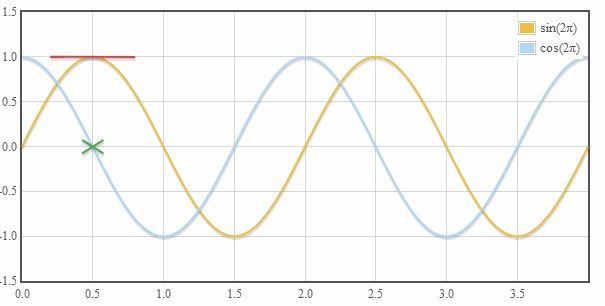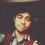# Weirdness is in graphs!As you all already know how the graph of $f\left(x\right)=\frac{\sin x}{x}$ looks like, try graphing $f\left(x\right)=\frac{\sin \left(\frac{1}{\left(x\right)}\right)}{\frac{1}{x}}$. (Yes! You're allowed to graph it using an electronic graphing calculator, or use the Desmos Graphing Calculator.)

Try graphing the following too!

$\tan \left(\frac{x}{\cos \left(\frac{1}{x}\right)}\right)$

$\tan \left(\frac{x}{\left(\sec x\right)}\right)$. (Nothing? Zoom out for the climax!)

The bombshell:

$\sin \left(\cos \left(\tan \left(\csc \left(\sec \left(\cot \left(\arcsin \left(\arccos \left(\arctan \left(\operatorname{arccsc}\left(\operatorname{arcsec}\left(\operatorname{arccot}\left(x\right)\right)\right)\right)\right)\right)\right)\right)\right)\right)\right)\right)$

And your device starts lagging a bit too as you move towards the negative x-axis.

$\frac{e^x}{\ln \left(\sin \left(e^{\cos \left(\frac{1}{\left(\left(\left(x\right)\right)\right)}\right)}\right)\right)}$

Seems as if the curve is a fan of the negative y-axis!

$\sin \left(\frac{1}{e^{\frac{1}{\operatorname{arccsc}\left(x\right)}}}\right)$

Kindly move towards the negative x-axis.

$y^x=\sec \left(20x\right)$

Clearly, this time the graphing calculator gave up!

You're free to share any such function which has such a peculiar curve in the comments section. Cheers! :D

GIF Credit: RedditNote by Arkajyoti Banerjee
4 years, 11 months ago

This discussion board is a place to discuss our Daily Challenges and the math and science related to those challenges. Explanations are more than just a solution — they should explain the steps and thinking strategies that you used to obtain the solution. Comments should further the discussion of math and science.

When posting on Brilliant:

• Use the emojis to react to an explanation, whether you're congratulating a job well done , or just really confused .
• Ask specific questions about the challenge or the steps in somebody's explanation. Well-posed questions can add a lot to the discussion, but posting "I don't understand!" doesn't help anyone.
• Try to contribute something new to the discussion, whether it is an extension, generalization or other idea related to the challenge.

MarkdownAppears as
*italics* or _italics_ italics
**bold** or __bold__ bold
- bulleted- list
• bulleted
• list
1. numbered2. list
1. numbered
2. list
Note: you must add a full line of space before and after lists for them to show up correctly
paragraph 1paragraph 2

paragraph 1

paragraph 2

[example link](https://brilliant.org)example link
> This is a quote
This is a quote
    # I indented these lines
# 4 spaces, and now they show
# up as a code block.

print "hello world"
# I indented these lines
# 4 spaces, and now they show
# up as a code block.

print "hello world"
MathAppears as
Remember to wrap math in $$ ... $$ or $ ... $ to ensure proper formatting.
2 \times 3 $2 \times 3$
2^{34} $2^{34}$
a_{i-1} $a_{i-1}$
\frac{2}{3} $\frac{2}{3}$
\sqrt{2} $\sqrt{2}$
\sum_{i=1}^3 $\sum_{i=1}^3$
\sin \theta $\sin \theta$
\boxed{123} $\boxed{123}$

Sort by:

Weird weird weird!!!

- 4 years, 11 months ago